1. /
2. CBSE
3. /
4. Class 07
5. /
6. Mathematics
7. /
8. NCERT Solutions for Class...

# NCERT Solutions for Class 7 Maths Exercise 5.1### myCBSEguide App

Download the app to get CBSE Sample Papers 2023-24, NCERT Solutions (Revised), Most Important Questions, Previous Year Question Bank, Mock Tests, and Detailed Notes.

NCERT solutions for Maths Lines and Angles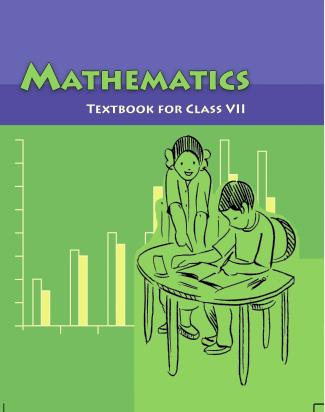## NCERT Solutions for Class 7 Maths Lines and Angles

###### Question 1.Find the complement of each of the following angles: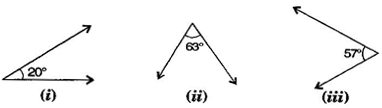Complementary angle = {tex}{90^ \circ } – {/tex} given angle

(i) Complement of {tex}{20^ \circ }{/tex} = {tex}{90^ \circ } – {20^ \circ } = {70^ \circ }{/tex}

(ii) Complement of {tex}{63^ \circ }{/tex} = {tex}{90^ \circ } – {63^ \circ } = {27^ \circ }{/tex}

Complement of {tex}{57^ \circ }{/tex} = {tex}{90^ \circ } – {57^ \circ } = {33^ \circ }{/tex}

NCERT Solutions for Class 7 Maths Exercise 5.1

###### Question 2.Find the supplement of each of the following angles: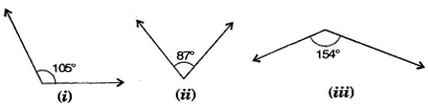Supplementary angle = {tex}{180^ \circ } – {/tex} given angle

(i) Supplement of {tex}{105^ \circ }{/tex} = {tex}{180^ \circ } – {105^ \circ } = {75^ \circ }{/tex}

(ii) Supplement of {tex}{87^ \circ }{/tex} = {tex}{180^ \circ } – {87^ \circ } = {93^ \circ }{/tex}

Supplement of {tex}{154^ \circ }{/tex} = {tex}{180^ \circ } – {154^ \circ } = {26^ \circ }{/tex}

NCERT Solutions for Class 7 Maths Exercise 5.1

###### Question 3.Identify which of the following pairs of angles are complementary and which are supplementary:

(i) {tex}65^\circ ,115^\circ {/tex}

(ii) {tex}63^\circ ,27^\circ {/tex}

(iii) {tex}112^\circ ,68^\circ {/tex}

(iv) {tex}130^\circ ,50^\circ {/tex}

(v) {tex}45^\circ ,45^\circ {/tex}

(vi) {tex}80^\circ ,10^\circ {/tex}

If sum of two angles is {tex}{180^ \circ }{/tex}, then they are called supplementary angles.

If sum of two angles is {tex}{90^ \circ },{/tex} then they are called complementary angles.

(i) {tex}65^\circ + 115^\circ = 180^\circ {/tex} These are supplementary angles.

(ii) {tex}63^\circ + 27^\circ = 90^\circ {/tex} These are complementary angles.

(iii) {tex}112^\circ + 68^\circ = 180^\circ {/tex} These are supplementary angles.

(iv) {tex}130^\circ + 50^\circ = 180^\circ {/tex} These are supplementary angles.

(v) {tex}{45^ \circ } + {45^ \circ } = {90^ \circ }{/tex} These are complementary angles.

(vi) {tex}80^\circ + 10^\circ = 90^\circ {/tex} These are complementary angles.

NCERT Solutions for Class 7 Maths Exercise 5.1

###### Question 4.Find the angle which is equal to its complement:

Let one of the two equal complementary angles be {tex}x.{/tex}

{tex}\therefore {/tex} {tex}x + x = {90^ \circ }{/tex} {tex} \Rightarrow {/tex} {tex}2x = {90^ \circ }{/tex}{tex} \Rightarrow {/tex} {tex}x = \frac{{{{90}^ \circ }}}{2} = {45^ \circ }{/tex}

Thus, {tex}{45^ \circ }{/tex} is equal to its complement.

NCERT Solutions for Class 7 Maths Exercise 5.1

###### Question 5.Find the angle which is equal to its supplement.

Let {tex}x{/tex} be two equal angles of its supplement.

Therefore, {tex}x + x = 180^\circ {/tex} [Supplementary angles]

{tex} \Rightarrow {/tex} {tex}2x = 180^\circ {/tex}

{tex} \Rightarrow {/tex} {tex}x = \frac{{180^\circ }}{2} = {90^ \circ }{/tex}

Thus, {tex}{90^ \circ }{/tex} is equal to its supplement.

NCERT Solutions for Class 7 Maths Exercise 5.1

###### Question 6.In the given figure, {tex}\angle {/tex}1 and {tex}\angle {/tex}2 are supplementary angles. If {tex}\angle {/tex}1 is decreased, what changes should take place in {tex}\angle {/tex}2 so that both the angles still remain supplementary?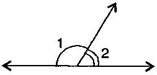I f{tex}\angle {/tex}1 is decreased then, {tex}\angle {/tex}2 will increase with the same measure, so that both the angles still remain supplementary.

NCERT Solutions for Class 7 Maths Exercise 5.1

###### Question 7.Can two angles be supplementary if both of them are:

(i) acute

(ii) obtuse

(iii) right?

(i) No, because sum of two acute angles is less than {tex}180^\circ .{/tex}

(ii) No, because sum of two obtuse angles is more than {tex}180^\circ .{/tex}

(iii) Yes, because sum of two right angles is {tex}180^\circ .{/tex}

NCERT Solutions for Class 7 Maths Exercise 5.1

###### Question 8. An angle is greater than {tex}{45^ \circ }.{/tex} Is its complementary angle greater than {tex}{45^ \circ }{/tex} or equal to {tex}{45^ \circ }{/tex} or less than {tex}{45^ \circ }?{/tex}

Let the complementary angles be {tex}x{/tex} and {tex}y,{/tex} i.e., {tex}x + y = {90^ \circ }{/tex}

It is given that {tex}x > {45^ \circ }{/tex}

Adding {tex}y{/tex} both sides, {tex}x + y > {45^ \circ } + y{/tex}

{tex} \Rightarrow {/tex} {tex}{90^ \circ } > {45^ \circ } + y{/tex} {tex} \Rightarrow {/tex} {tex}{90^ \circ } – {45^ \circ } > y{/tex}{tex} \Rightarrow {/tex} {tex}y < {45^ \circ }{/tex}

Thus, its complementary angle is less than {tex}{45^ \circ }.{/tex}

NCERT Solutions for Class 7 Maths Exercise 5.1

###### Question 9.In the adjoining figure: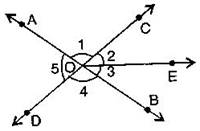1. Is {tex}\angle {/tex}1 adjacent to {tex}\angle {/tex}2?
2. Is {tex}\angle {/tex}AOC adjacent to {tex}\angle {/tex}AOE?
3. Do {tex}\angle {/tex}COE and {tex}\angle {/tex}EOD form a linear pair?
4. Are {tex}\angle {/tex}BOD and {tex}\angle {/tex}DOA supplementary?
5. Is {tex}\angle {/tex}1 vertically opposite to {tex}\angle {/tex}4?
6. What is the vertically opposite angle of {tex}\angle {/tex}5?

(i) Yes, in {tex}\angle {/tex}AOE, OC is common arm.

(ii) No, they have no non-common arms on opposite side of common arm.

(iii) Yes, they form linear pair.

(iv) Yes, they are supplementary.

(v) Yes, they are vertically opposite angles.

(vi) Vertically opposite angles of {tex}\angle {/tex}5 is {tex}\angle {/tex}COB.

NCERT Solutions for Class 7 Maths Exercise 5.1

###### Question 10.Indicate which pairs of angles are: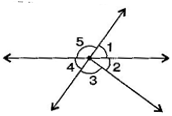1. Vertically opposite angles?
2. Linear pairs?

(i) Vertically opposite angles, {tex}\angle {/tex}1, {tex}\angle {/tex}4; {tex}\angle {/tex}5, {tex}\angle {/tex}2 + {tex}\angle {/tex}3.

(ii) Linear pairs {tex}\angle {/tex}1, {tex}\angle {/tex}5; {tex}\angle {/tex}5, {tex}\angle {/tex}4.

Question 11.In the following figure, is {tex}\angle {/tex}1 adjacent to {tex}\angle {/tex}2? Give reasons.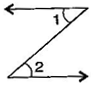{tex}\angle {/tex}1 and {tex}\angle {/tex}2 are not adjacent angles because their vertex is not common.

###### Question 12.Find the values of the angles {tex}x,y{/tex} and {tex}z{/tex} in each of the following: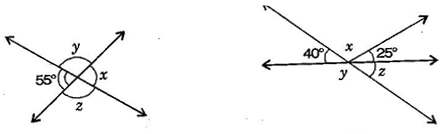(i) {tex}x = 55^\circ {/tex} [Vertically opposite angles]

Now {tex}55^\circ + y = 180^\circ {/tex} [Linear pair]

{tex} \Rightarrow {/tex} {tex}y = 180^\circ – 55^\circ = 125^\circ {/tex}

Also {tex}y = z = 125^\circ {/tex} [Vertically opposite angles]

Thus, {tex}x = 55^\circ ,y = 125^\circ {/tex} and {tex}z = 125^\circ .{/tex}

(ii) {tex}40^\circ + x + 25^\circ = 180^\circ {/tex} [Angles on straight line]

{tex} \Rightarrow {/tex} {tex}65^\circ + x = 180^\circ {/tex}

{tex} \Rightarrow {/tex} {tex}x = 180^\circ – 65^\circ {/tex} = {tex}115^\circ {/tex}

Now {tex}40^\circ + y = 180^\circ {/tex} [Linear pair]

{tex} \Rightarrow {/tex} {tex}y = 180^\circ – 40^\circ = 140^\circ {/tex} ……….(i)

Also {tex}y + z = 180^\circ {/tex} [Linear pair]

{tex} \Rightarrow {/tex} {tex}140^\circ + z = 180^\circ {/tex} [From eq. (i)]

{tex} \Rightarrow {/tex} {tex}z = 180^\circ – 140^\circ = 40^\circ {/tex}

Thus, {tex}x = 115^\circ ,y = 140^\circ {/tex} and {tex}z = 40^\circ .{/tex}

NCERT Solutions for Class 7 Maths Exercise 5.1

###### Question 13.Fill in the blanks:
1. If two angles are complementary, then the sum of their measures is _______________.
2. If two angles are supplementary, then the sum of their measures is _______________.
3. Two angles forming a linear pair are _______________.
4. If two adjacent angles are supplementary, they form a _______________.
5. If two lines intersect a point, then the vertically opposite angles are always _______________.
6. If two lines intersect at a point and if one pair of vertically opposite angles are acute angles, then the other pair of vertically opposite angles are _______________.

(i) {tex}{90^ \circ }{/tex}

(ii) {tex}180^\circ {/tex}

(iii) supplementary

(iv) linear pair

(v) equal

(vi) obtuse angles

NCERT Solutions for Class 7 Maths Exercise 5.1

###### Question 14.In the adjoining figure, name the following pairs of angles: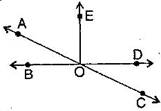1. Obtuse vertically opposite angles.
3. Equal supplementary angles.
4. Unequal supplementary angles.
5. Adjacent angles that do not form a linear pair.

(i) Obtuse vertically opposite angles means greater than {tex}{90^ \circ }{/tex} and equal {tex}\angle {/tex}AOD = {tex}\angle {/tex}BOC.

(ii) Adjacent complementary angles means angles have common vertex, common arm, non-common arms are on either side of common arm and sum of angles is {tex}{90^ \circ }.{/tex}

(iii) Equal supplementary angles means sum of angles is {tex}180^\circ {/tex} and supplement angles are equal.

(iv) Unequal supplementary angles means sum of angles is {tex}180^\circ {/tex} and supplement angles are unequal.

i.e., {tex}\angle {/tex}AOE, {tex}\angle {/tex}EOC; {tex}\angle {/tex}AOD, {tex}\angle {/tex}DOC and {tex}\angle {/tex}AOB, {tex}\angle {/tex}BOC

(v) Adjacent angles that do not form a linear pair mean, angles have common ray but the angles in a linear pair are not supplementary.

i.e., {tex}\angle {/tex}AOB, {tex}\angle {/tex}AOE; {tex}\angle {/tex}AOE, {tex}\angle {/tex}EOD and {tex}\angle {/tex}EOD, {tex}\angle {/tex}COD

## NCERT Solutions for Class 7 Maths Exercise 5.1

NCERT Solutions Class 7 Maths PDF (Download) Free from myCBSEguide app and myCBSEguide website. Ncert solution class 7 Maths includes text book solutions from Class 7 Maths Book . NCERT Solutions for CBSE Class 7 Maths have total 15 chapters. 7 Maths NCERT Solutions in PDF for free Download on our website. Ncert Maths class 7 solutions PDF and Maths ncert class 7 PDF solutions with latest modifications and as per the latest CBSE syllabus are only available in myCBSEguide.

## CBSE app for Students

To download NCERT Solutions for Class 7 Maths, Social Science Computer Science, Home Science, Hindi English, Maths Science do check myCBSEguide app or website. myCBSEguide provides sample papers with solution, test papers for chapter-wise practice, NCERT solutions, NCERT Exemplar solutions, quick revision notes for ready reference, CBSE guess papers and CBSE important question papers. Sample Paper all are made available through the best app for CBSE students and myCBSEguide website.### Test Generator

Create question paper PDF and online tests with your own name & logo in minutes.### myCBSEguide

Question Bank, Mock Tests, Exam Papers, NCERT Solutions, Sample Papers, Notes

### 8 thoughts on “NCERT Solutions for Class 7 Maths Exercise 5.1”

1. Thanks

2. It is very important for me to be able to learn the math

Thank you ?????????????????????????????

3. nice website

4. Thank you!….

5. Thanks I am very thankful to you

6. Thanks My school homework is complete

7. nice website and with this I can easily learn maths for my exams than you and update like this more so it will be very useful for us who are seeing this website

8. Thanku my home work completed